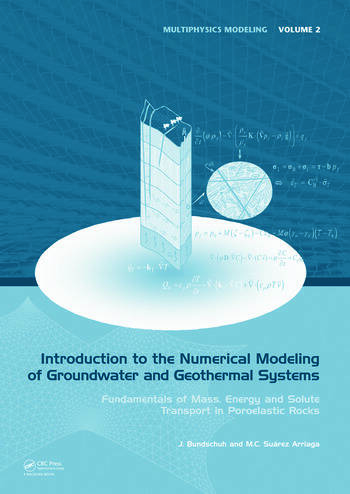Introduction to the Numerical Modeling of Groundwater and Geothermal Systems: Fundamentals of Mass, Energy and Solute Transport in Poroelastic Rocks

1st Edition

Jochen Bundschuh, Mario César Suárez A.

CRC Press
Published July 5, 2010
Reference - 522 Pages
ISBN 9780415401678 - CAT# SW1674
Series: Multiphysics Modeling

For Instructors Request Inspection Copy

was \$275.00

USD\$220.00

SAVE ~\$55.00

FREE Standard Shipping!

Preview

Summary

This book provides an introduction to the scientific fundamentals of groundwater and geothermal systems. In a simple and didactic manner the different water and energy problems existing in deformable porous rocks are explained as well as the corresponding theories and the mathematical and numerical tools that lead to modeling and solving them. This approach provides the reader with a thorough understanding of the basic physical laws of thermoporoelastic rocks, the partial differential equations representing these laws and the principal numerical methods, which allow finding approximate solutions of the corresponding mathematical models. The book also presents the form in which specific useful models can be generated and solved. The text is introductory in the sense that it explains basic themes of the systems mentioned in three areas: engineering, physics and mathematics. All the laws and equations introduced in this book are formulated carefully based on fundamental physical principles. This way, the reader will understand the key importance of mathematics applied to all the subjects. Simple models are emphasized and solved with numerous examples. For more sophisticated and advanced models the numerical techniques are described and developed carefully.
This book will serve as a synoptic compendium of the fundamentals of fluid, solute and heat transport, applicable to all types of subsurface systems, ranging from shallow aquifers down to deep geothermal reservoirs.
The book will prove to be a useful textbook to senior undergraduate and graduate students, postgraduates, professional geologists and geophysicists, engineers, mathematicians and others working in the vital areas of groundwater and geothermal resources.

Instructors

We provide complimentary e-inspection copies of primary textbooks to instructors considering our books for course adoption.